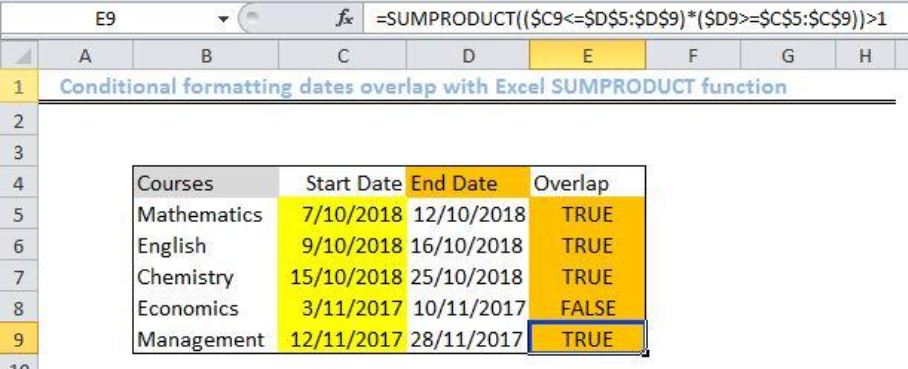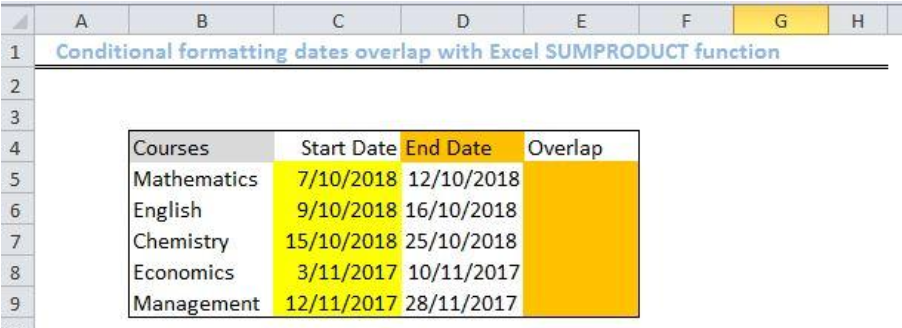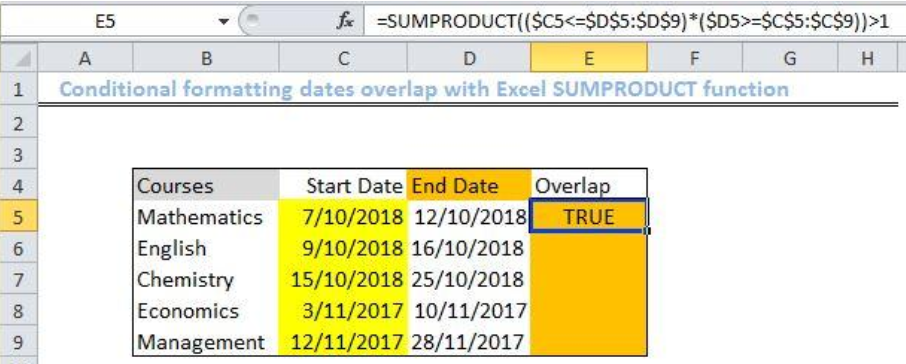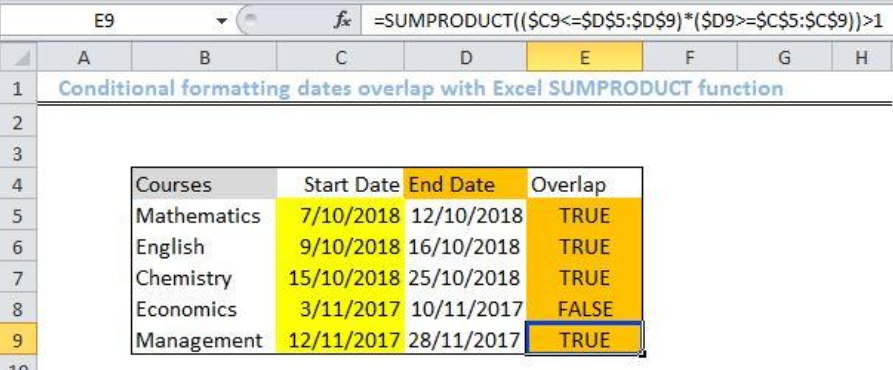Get instant live expert help with Excel or Google Sheets“My Excelchat expert helped me in less than 20 minutes, saving me what would have been 5 hours of work!”

#### Post your problem and you’ll get Expert help in seconds.

Your message must be at least 40 characters
Our professional Expert are available now. Your privacy is guaranteed.

# Conditional formatting dates overlap

We can use the Excel SUMPRODUCT function to check conditional formatting dates overlap in an array. In easy steps, we will teach you how to use this function to handle array comparisons seamlessly.Figure 1: Result of using the Excel SUMPRODUCT function to check conditional formatting dates overlap

## General Formula

`=SUMPRODUCT((start_date<=end_dates)*(end_date>=start_dates))>1`

## Formula

`=SUMPRODUCT((\$C5<=\$D\$5:\$D\$9)*(\$D5>=\$C\$5:\$C\$9))>1`

## Setting up the Data

• We will set up a list of Courses in Column B
• Column C will contain the list of Start Dates for these courses
• We will input the End date for our list of Courses in Column D
• Column E will be titled Overlap. This is where we will insert the formula to check conditional formatting overlap and return the result as TRUE or FALSE.Figure 2: Setting up the Data

## Applying the Excel SUMPRODUCT function

• We will click on Cell E5
• We will insert the formula below into Cell E5
`=SUMPRODUCT((\$C5<=\$D\$5:\$D\$9)*(\$D5>=\$C\$5:\$C\$9))>1`
• We will press the enter keyFigure 3: Using the Excel SUMPRODUCT function

• We click again on Cell E5 and use the fill handle tool (a plus sign found at the base of Cell E5) to drag down the formula into the other cells.Figure 4: Result of applying the SUMPRODUCT function to check conditional formatting dates overlap in Excel

## Explanation

`=SUMPRODUCT((\$C5<=\$D\$5:\$D\$9)*(\$D5>=\$C\$5:\$C\$9))>1`

The Excel SUMPRODUCT function can multiply ranges or arrays together to return with the sum of products. In our above illustration, we used the Excel SUMPRODUCT function to highlight cells where their dates overlap. It literally checks the start and end date of each course, against all start and end dates in our excel sheet respectively. However, this formula depends on two conditions to return with a TRUE result:

• The Start Date must be less than or equal (<=) to at least one other end date and the entire list or range.
• The End Date must be greater than or equal to (>=) to at least one other date in the data.

The formula works by:

• Checking a Course Start Date against the other End Dates using the expression `(\$C5<=\$D\$5:\$D\$9)`. This will return with an outcome like this: `{1,1,1,1,1}`.
• Next, it checks the Course start date against other start dates using the expression `(\$D5>=\$C\$5:\$C\$9)`. This will return with an outcome like this: `{1,1,0,0,0}`.

It is solved like this:

`=SUMPRODUCT({0;1;1;1;1}*{1;1;1;0;0})>1`

`=SUMPRODUCT({0;1;1;0;0})>1`

`=TRUE`

## Instant Connection to an Expert through our Excelchat Service

Most of the time, the problem you will need to solve will be more complex than a simple application of a formula or function. If you want to save hours of research and frustration, try our live Excelchat service! Our Excel Experts are available 24/7 to answer any Excel question you may have. We guarantee a connection within 30 seconds and a customized solution within 20 minutes.

### Did this post not answer your question? Get a solution from connecting with the expert.Another blog reader asked this question today on Excelchat:## Subscribe to Excelchat.coAnother blog reader asked this question today on Excelchat: